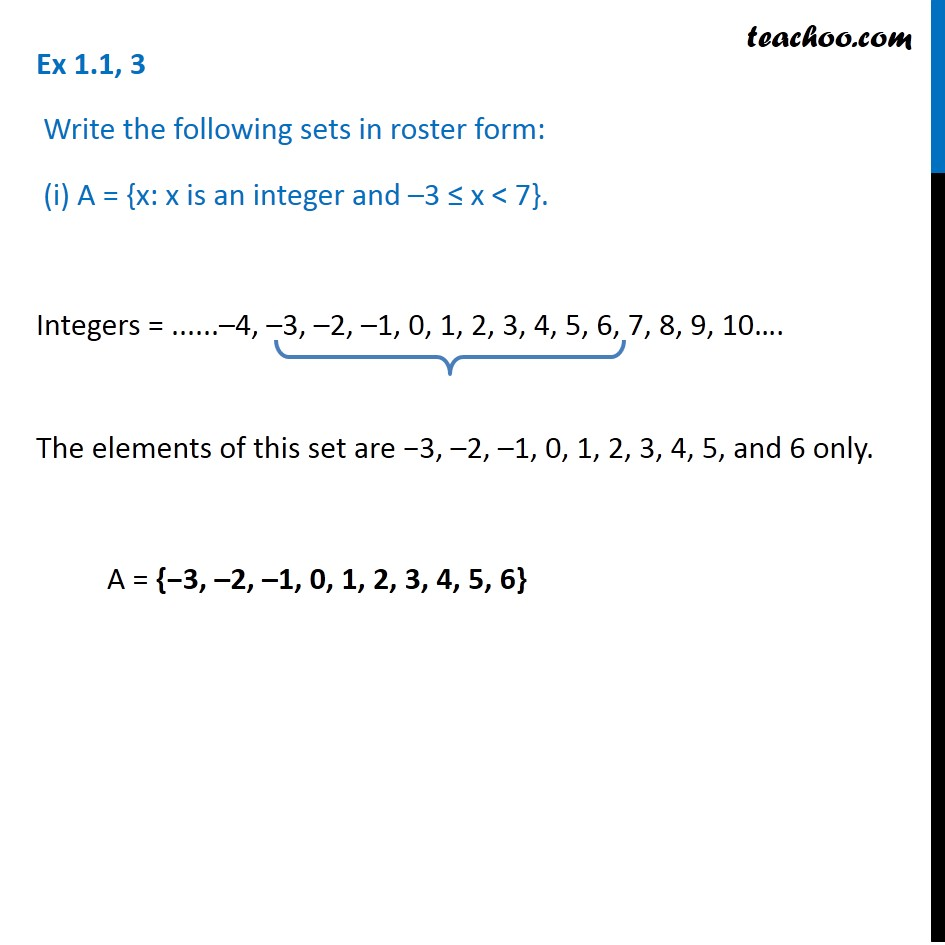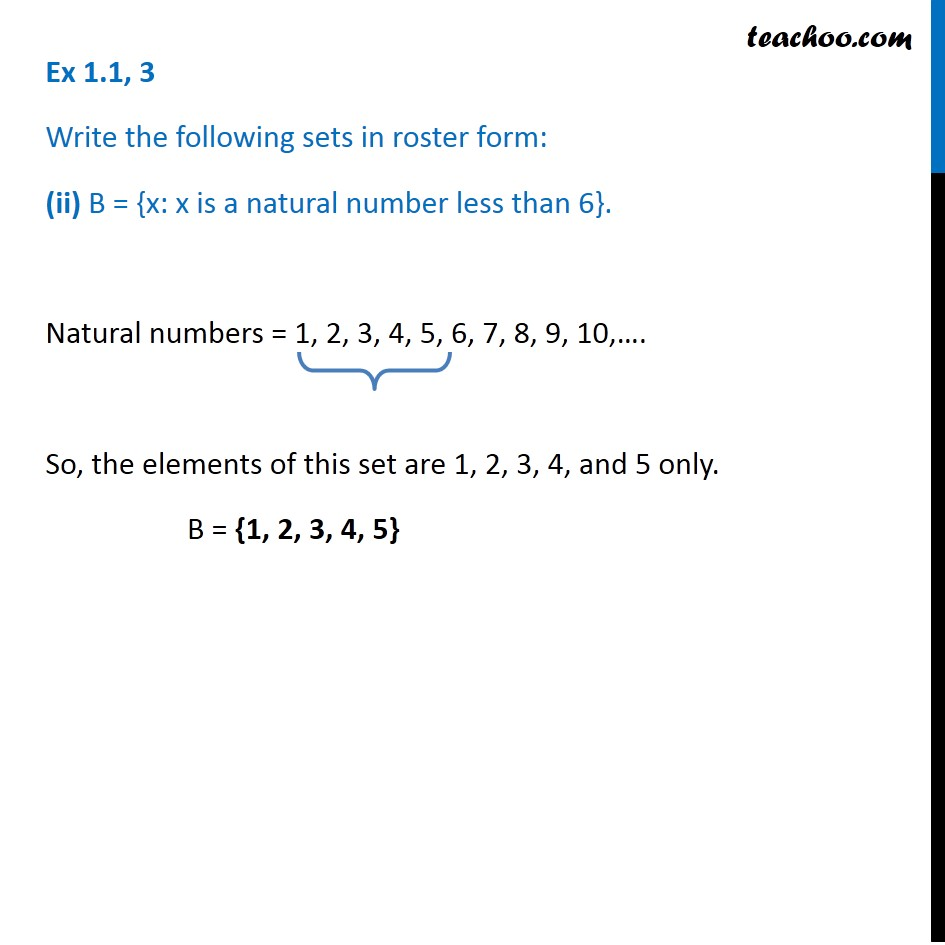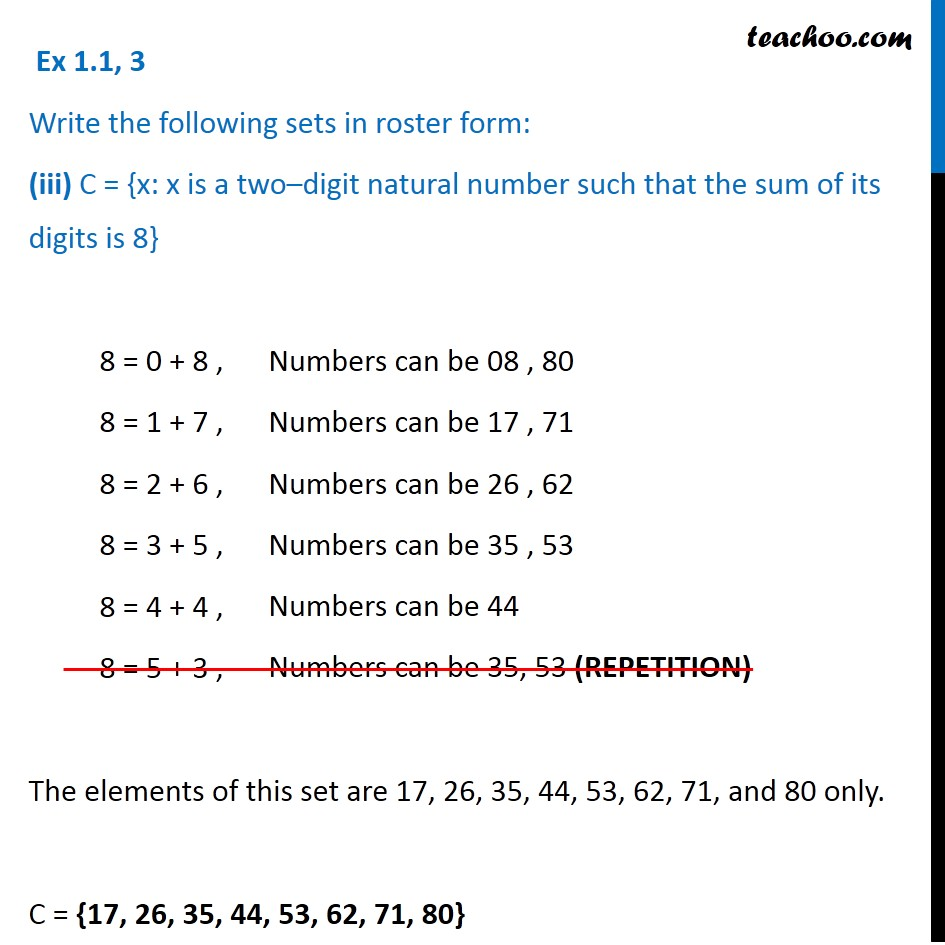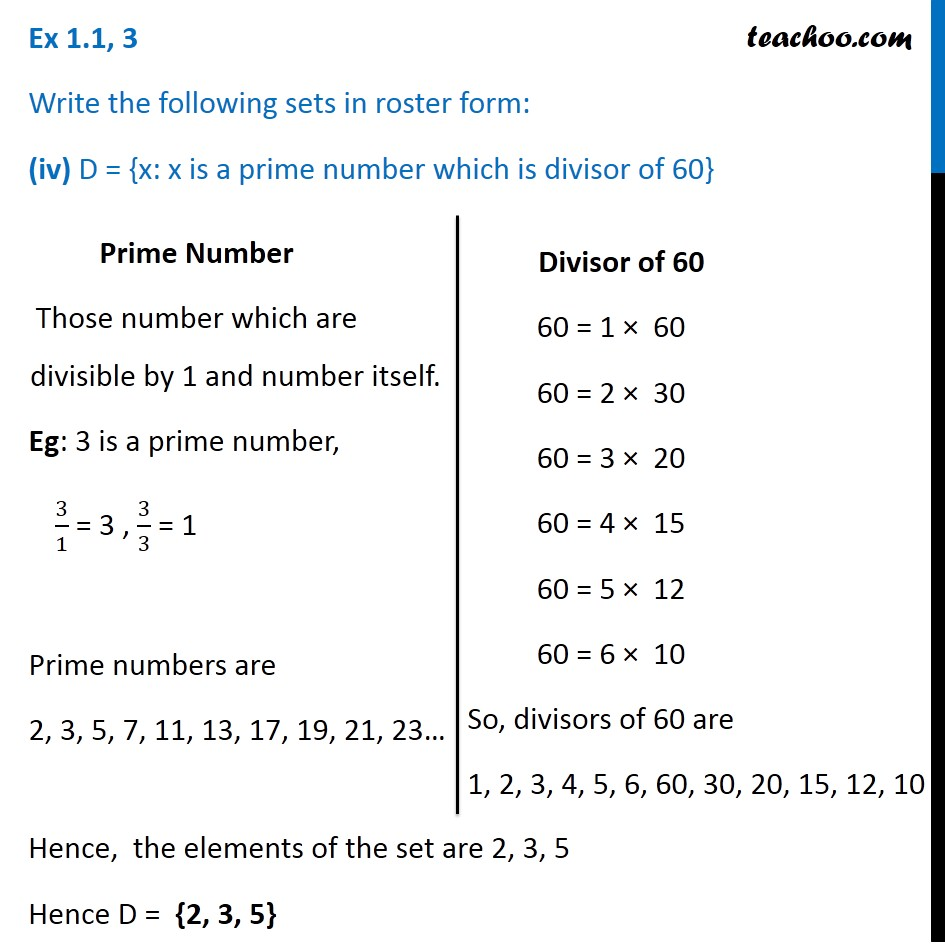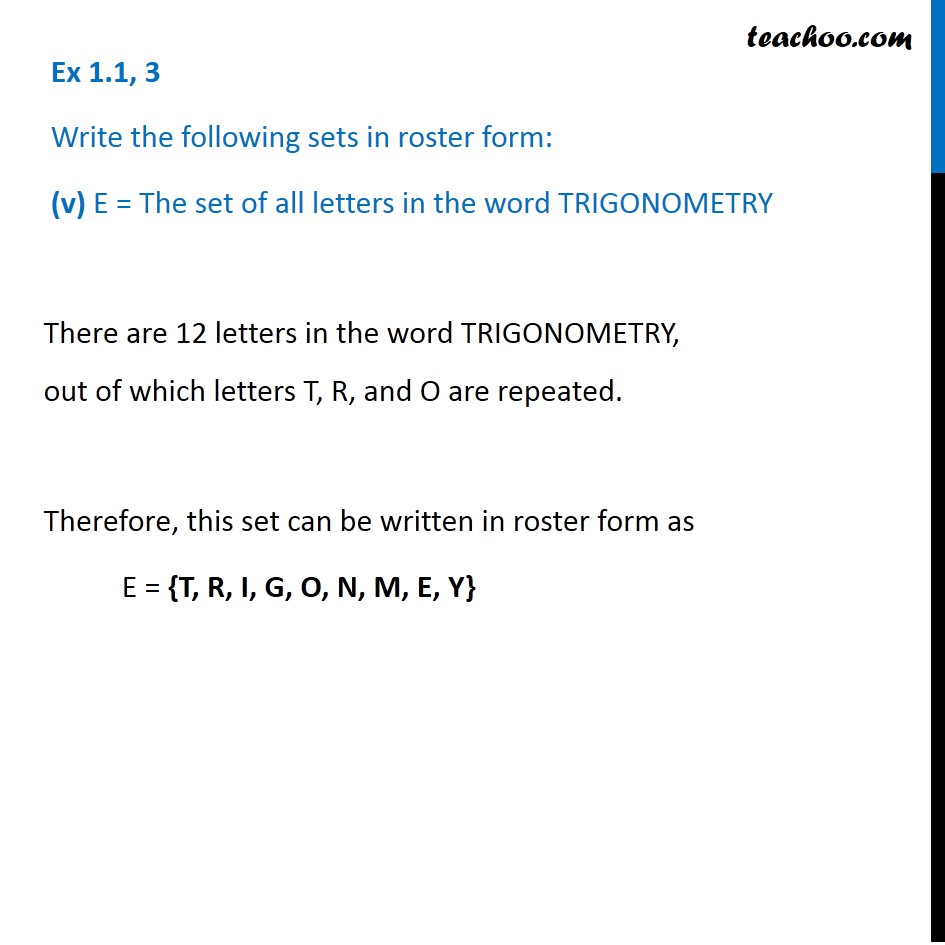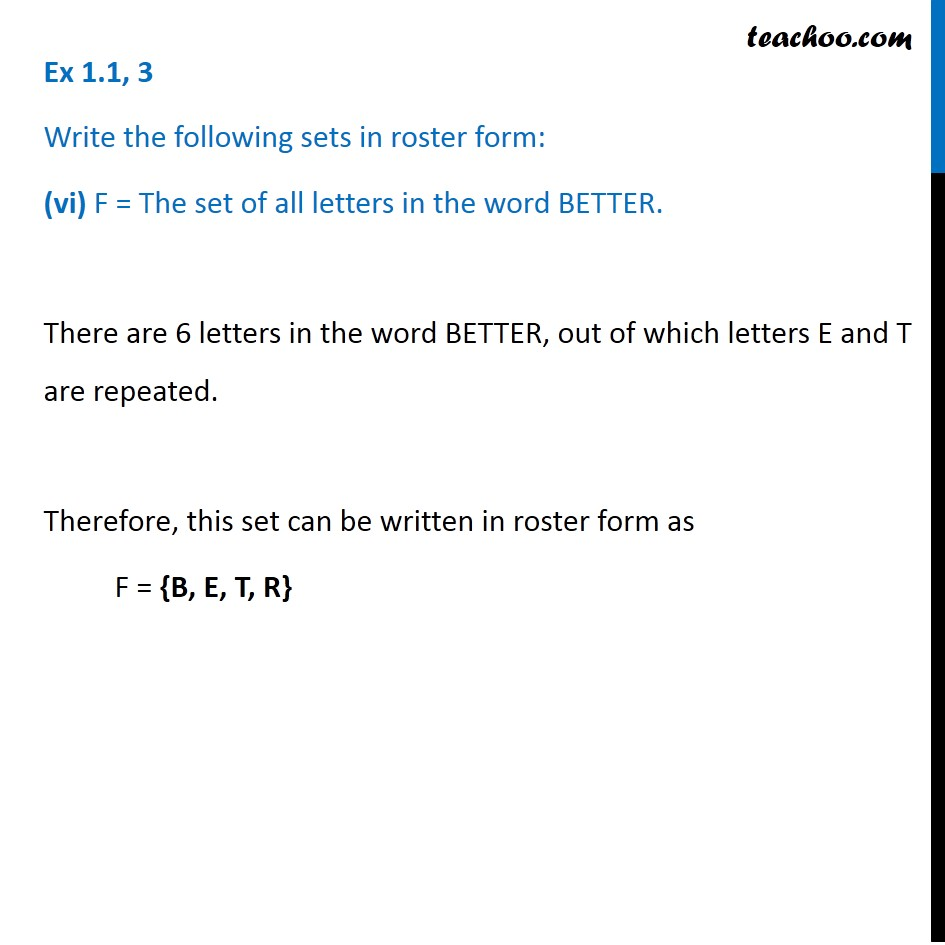1. Chapter 1 Class 11 Sets
2. Serial order wise
3. Ex 1.1

Transcript

Ex 1.1, 3 Write the following sets in roster form: (i) A = {x: x is an integer and –3 ﻿≤ x < 7}. Integers = ......–4, –3, –2, –1, 0, 1, 2, 3, 4, 5, 6, 7, 8, 9, 10…. The elements of this set are −3, –2, –1, 0, 1, 2, 3, 4, 5, and 6 only. A = {−3, –2, –1, 0, 1, 2, 3, 4, 5, 6} Ex 1.1, 3 Write the following sets in roster form: (ii) B = {x: x is a natural number less than 6}. Natural numbers = 1, 2, 3, 4, 5, 6, 7, 8, 9, 10,…. So, the elements of this set are 1, 2, 3, 4, and 5 only. B = {1, 2, 3, 4, 5} Ex 1.1, 3 Write the following sets in roster form: (iii) C = {x: x is a two–digit natural number such that the sum of its digits is 8} 8 = 0 + 8 , 8 = 1 + 7 , 8 = 2 + 6 , 8 = 3 + 5 , 8 = 4 + 4 , 8 = 5 + 3 , The elements of this set are 17, 26, 35, 44, 53, 62, 71, and 80 only. C = {17, 26, 35, 44, 53, 62, 71, 80} Ex 1.1, 3 Write the following sets in roster form: (iv) D = {x: x is a prime number which is divisor of 60} Hence, the elements of the set are 2, 3, 5 Hence D = {2, 3, 5} Prime Number Those number which are divisible by 1 and number itself. Eg: 3 is a prime number, 3/1 = 3 , 3/3 = 1 Prime numbers are 2, 3, 5, 7, 11, 13, 17, 19, 21, 23… Divisor of 60 60 = 1 × 60 60 = 2 × 30 60 = 3 × 20 60 = 4 × 15 60 = 5 × 12 60 = 6 × 10 So, divisors of 60 are 1, 2, 3, 4, 5, 6, 60, 30, 20, 15, 12, 10 Ex 1.1, 3 Write the following sets in roster form: (vi) F = The set of all letters in the word BETTER. There are 6 letters in the word BETTER, out of which letters E and T are repeated. Therefore, this set can be written in roster form as F = {B, E, T, R}

Ex 1.1

Chapter 1 Class 11 Sets
Serial order wise